# Matrix Algebra

## Producer Field Guide

HGD_Product
Producer Field Guide
HGD_Portfolio_Suite
Producer

A matrix is a set of numbers or values arranged in a rectangular array. If a matrix has i rows and j columns, it is said to be an i by j matrix.

A one-dimensional matrix, having one column (i by 1) is one of many kinds of vectors. For example, the measurement vector of a pixel is an n-element vector of the data file values of the pixel, where n is equal to the number of bands.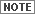See Enhancement for information on eigenvectors.

Matrix Notation

Matrices and vectors are usually designated with a single capital letter, such as M. For example: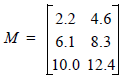One value in the matrix M would be specified by its position, which is its row and column (in that order) in the matrix. One element of the array (one value) is designated with a lower case letter and its position:

m3,2 = 12.4

With column vectors, it is simpler to use only one number to designate the position: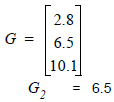Matrix Multiplication

A simple example of the application of matrix multiplication is a 1st-order transformation matrix. The coefficients are stored in a 2 × 3 matrix: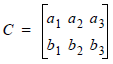Then, where: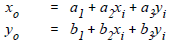xi and yi = source coordinates

xo and yo = rectified coordinates

The coefficients of the transformation matrix are as above.

The above could be expressed by a matrix equation: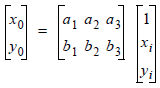or

R = CS

Where:

R = matrix of rectified coordinates (2 by 1)

C = transformation matrix (2 by 3)

S = a matrix of the source coordinates (3 by 1)

The sizes of the matrices are shown above to demonstrate a rule of matrix multiplication. To multiply two matrices, the first matrix must have the same number of columns as the second matrix has rows. For example, if the first matrix is a by b, and the second matrix is m by n, then b must equal m, and the product matrix has the size a by n.

The formula for multiplying two matrices is: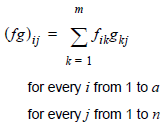Where:

i = a row in the product matrix

j = a column in the product matrix

f = an (a by b) matrix

g = an (m by n) matrix (b must equal m)

fg is an a by n matrix.

Transposition

The transposition of a matrix is derived by interchanging its rows and columns. Transposition is denoted by T, as in the following example (Cullen, 1972).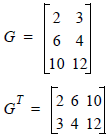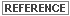For more information on transposition, see Computing Principal Components and Classification Decision Rules.

Trace

The trace of a square matrix is the sum of the elements of its main diagonal.

For example, given the following 3x3 matrix: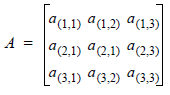The trace of A is given by: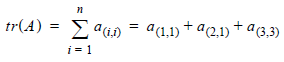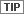Trace applies to square matrices only.